Hostname: page-component-797576ffbb-42xl8 Total loading time: 0 Render date: 2023-12-06T12:20:47.843Z Has data issue: false Feature Flags: { "corePageComponentGetUserInfoFromSharedSession": true, "coreDisableEcommerce": false, "useRatesEcommerce": true } hasContentIssue false

# Scott topology on Smyth power posets

Published online by Cambridge University Press:  27 July 2023

## Abstract

For a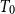$T_0$ space X, let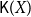$\mathsf{K}(X)$ be the poset of all nonempty compact saturated subsets of X endowed with the Smyth order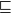$\sqsubseteq$.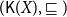$(\mathsf{K}(X), \sqsubseteq)$ (shortly$\mathsf{K}(X)$) is called the Smyth power poset of X. In this paper, we mainly discuss some basic properties of the Scott topology on Smyth power posets. It is proved that for a well-filtered space X, its Smyth power poset$\mathsf{K}(X)$ with the Scott topology is still well-filtered, and a$T_0$ space Y is well-filtered iff the Smyth power poset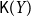$\mathsf{K}(Y)$ with the Scott topology is well-filtered and the upper Vietoris topology is coarser than the Scott topology on$\mathsf{K}(Y)$. A sober space Z is constructed for which the Smyth power poset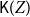$\mathsf{K}(Z)$ with the Scott topology is not sober. A few sufficient conditions are given for a$T_0$ space X under which its Smyth power poset$\mathsf{K}(X)$ with the Scott topology is sober. Some other properties, such as local compactness, first-countability, Rudin property and well-filtered determinedness, of Smyth power spaces, and the Scott topology on Smyth power posets, are also investigated.

Type
Paper
Information
Mathematical Structures in Computer Science , October 2023 , pp. 832 - 867

## Access options

Get access to the full version of this content by using one of the access options below. (Log in options will check for institutional or personal access. Content may require purchase if you do not have access.)

## Footnotes

This research was supported by the National Natural Science Foundation of China (Nos. 12071199, 12071188, 11661057).

Dedicated to Professor Dana Scott on the occasion of his 90th birthday

## References

Abramsky, S. and Jung, A. (1994). Domain theory. In: Semantic Structures, Handbook of Logic in Computer Science, vol. 3, Warzawa, Polish Scientific Publishers, 1168.Google Scholar
Brecht, M. and Kawai, T. (2019). On the commutativity of the powerspace constructions. Logical Methods in Computer Science 15 (3) 13:113:25.Google Scholar
Engelking, R. (1989). General Topology, Warzawa, Polish Scientific Publishers.Google Scholar
Erné, M. (2009). Infinite distributive laws versus local connectedness and compactness properties. Topology and Its Applications 156 20542069.CrossRefGoogle Scholar
Erné, M. (2018). Categories of locally hypercompact spaces and quasicontinuous posets. Applied Categorical Structures 26 823854.CrossRefGoogle Scholar
Gierz, G., Hofmann, K., Keimel, K., Lawson, J., Mislove, M. and Scott, D. (2003). Continuous Lattices and Domains, Cambridge, Cambridge University Press.CrossRefGoogle Scholar
Gierz, G., Lawson, J. and Stralka, A. (1983). Quasicontinuous posets. Houston Journal of Mathematics 9 191208.Google Scholar
Goubault-Larrecq, J. (2012). QRB-domains and the probabilistic powerdomain. Logical Methods in Computer Science 8 (1:14) 133.Google Scholar
Goubault-Larrecq, J. (2013). Non-Hausdorff Topology and Domain Theory, New Mathematical Monographs, vol. 22, Cambridge, Cambridge University Press.Google Scholar
Heckmann, R. (1992). An upper power domain construction in terms of strongly compact sets. In: Mathematical Foundations of Programming Semantics, Lecture Notes in Computer Science, vol. 598, Berlin, Springer-Verlag, 272293.CrossRefGoogle Scholar
Heckmann, R. and Keimel, K. (2013). Quasicontinuous domains and the Smyth powerdomain. Electronic Notes in Theoretical Computer Science 298 215232.CrossRefGoogle Scholar
Hofmann, K. and Lawson, J. (1978). The spectral theory of distributive continuous lattices. Transactions of the Amercan Mathematical Society 246 285310.CrossRefGoogle Scholar
Isbell, J. (1982). Completion of a construction of Johnstone. Proccedings of the Amercan Mathematical Society 85 333334.CrossRefGoogle Scholar
Jia, X. (2018). Meet-Continuity and Locally Compact Sober Dcpos. Phd thesis, University of Birmingham.Google Scholar
Johnstone, P. (1981). Scott is not always sober. In: Continuous Lattices, Lecture Notes in Mathematics, vol. 871, Berlin, Springer-Verlag, 282283.CrossRefGoogle Scholar
Kou, H. (2001).$U_k$ -admitting dcpos need not be sober. In: Domains and Processes, Semantic Structure on Domain Theory, vol. 1, Netherlands, Kluwer Academic Publishers, 4150.Google Scholar
Lawson, J., Wu, G. and Xi, X. (2020). Well-filtered spaces, compactness, and the lower topology. Houston Journal of Mathematics 46 (1) 283294.Google Scholar
Lu, C. and Li, Q. (2017). Weak well-filtered spaces and coherence. Topology and Its Applications 230 373380.CrossRefGoogle Scholar
Lyu, Z., Chen, Y. and Jia, X. (2022). Core-compactness, consonance and the Smyth powerspaces. Topology and Its Applications 312 108066.CrossRefGoogle Scholar
Miao, M., Li, Q. and Zhao, D. (2021). On two problems about sobriety of topological spaces. Topology and Its Applications 295 107667.CrossRefGoogle Scholar
Plotkin, G. (1976). A powerdomain construction. SIAM Journal on Computing 5 452487.CrossRefGoogle Scholar
Plotkin, G. (1982). A powerdomain for countable non-determinism. In: International Conference on Automata, Languages and programming, Lecture Notes in Computer Science, vol. 140, Berlin, Springer-Verlag, 418428.CrossRefGoogle Scholar
Rudin, M. (1981). Directed sets which converge. In: General Topology and Modern Analysis, University of California, Riverside, 1980, Academic Press, 305307.Google Scholar
Schalk, A. (1993). Algebras for Generalized Power Constructions. Phd thesis, Technische Hochschule Darmstadt.Google Scholar
Shen, C., Xi, X., Xu, X. and Zhao, D. (2019). On well-filtered reflections of$T_0$ spaces. Topology and Its Applications 267 106869.CrossRefGoogle Scholar
Smyth, M. (1978). Power domains. Journal of Computer and System Sciences 16 2336.CrossRefGoogle Scholar
Wyler, U. (1981). Dedekind complete posets and Scott topologies. In: Continuous Lattices, Lecture Notes in Math, vol. 871, Berlin, Springer-Verlag, 384389.CrossRefGoogle Scholar
Xi, X. and Lawson, J. (2017). On well-filtered spaces and ordered sets. Topology and Its Applications 228 139144.CrossRefGoogle Scholar
Xu, X. (2021). On H-sober spaces and H-sobrifications of$T_{0}$ spaces. Topology and Its Applications 289 107548.CrossRefGoogle Scholar
Xu, X., Shen, C., Xi, X. and Zhao, D. (2020a). First countability,$\omega$ -well-filtered spaces and reflections. Topology and Its Applications 279 107255.CrossRefGoogle Scholar
Xu, X., Shen, C., Xi, X. and Zhao, D. (2020b). On$T_{0}$ spaces determined by well-filtered spaces. Topology and Its Applications 282 107323.CrossRefGoogle Scholar
Xu, X., Shen, C., Xi, X. and Zhao, D. (2021a). First-countability,$\omega$ -Rudin spaces and well-filtered determined. Topology and Its Applications 300 107775.CrossRefGoogle Scholar
Xu, X., Xi, X. and Zhao, D. (2021b). A complete Heyting algebra whose Scott topology is non-sober. Fundammenta Mathematicae 252 315323.CrossRefGoogle Scholar
Xu, X. and Yang, Z. (2021c). Coincidence of the upper Vietoris topology and the Scott topology. Topology and Its Applications 288 107480.CrossRefGoogle Scholar
Xu, X. and Zhao, D. (2021d). Some open problems on well-filtered spaces and sober spaces. Topology and Its Applications 301 107540.CrossRefGoogle Scholar
Zhao, D. and Ho, W. (2015). On topologies defined by irreducible sets. Journal of Logical and Algebraic Methods in Programming 84 (1) 185195.CrossRefGoogle Scholar